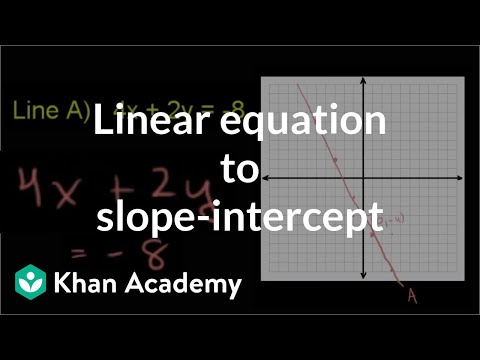Video

# Slope-intercept equation from slope & point (Full video)

Description: Learn how to find the equation of the line with a slope of -3/4 that goes through the point (0,8). Created by Sal Khan and Monterey Institute for Technology and Education. A line has a slope of negative 3/4 and goes through the point 0 comma 8. What is the equation of this line in slope-intercept form? So any line can be represented in slope-intercept form, is y is equal to mx plus b, where this m right over here, that is of the slope of the line.

### Other videos you might be interested in### Ratio and Proportion in Grades 6-8: Connections to College and Career Skills (Full video)

#### The Hunt Institute